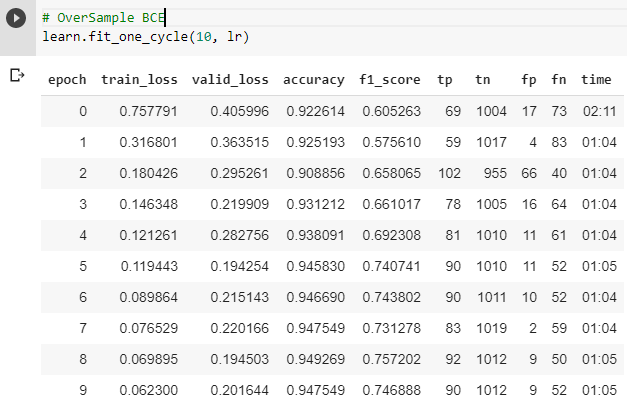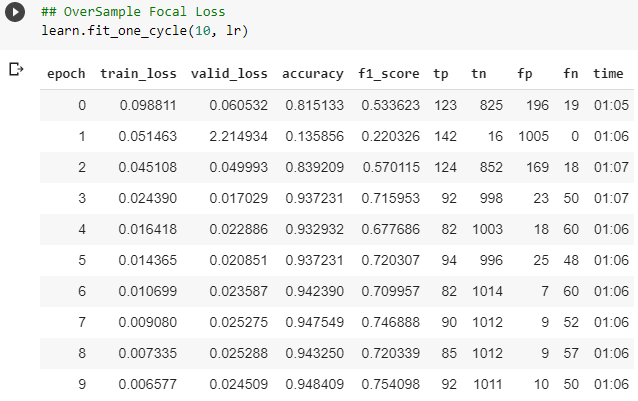# Highly imbalanced data

Hello!

I am working with a strongly imbalanced data where the real output is 98% category A and 2% of the time category B. I have a large dataset (20 million rows) for this classification problem. I am thinking of using oversampling for training but as detecting both classes right is very important, I fail to see which loss and metric might turn out to be most useful.
I am thinking of using Matthews correlation coefficient (MCC) as a metric and I don’t know if there is a loss that might be some kind of proxy to this metric or any other similar metric (F1 score, etc).
Would you mind sharing what you think might work well?

Thanks

1 Like

I would try `Focal Loss` and oversampling as you mentioned. But I would start with the default approach without oversampling and the default loss just to have a baseline for comparison.

Then you can look where the model fails and that may give some ideas for improving those cases.

I hope this helps4 Likes

I’m going to give it a try and reply with a feedback when done. Thanks !

are there implementations of Focal Loss or Oversampling in fastai?

Focal Loss (as posted here by bestfitting):

``````class FocalLoss(nn.Module):
def __init__(self, gamma=2):
super().__init__()
self.gamma = gamma

def forward(self, logit, target):
target = target.float()
max_val = (-logit).clamp(min=0)
loss = logit - logit * target + max_val + \
((-max_val).exp() + (-logit - max_val).exp()).log()

invprobs = F.logsigmoid(-logit * (target * 2.0 - 1.0))
loss = (invprobs * self.gamma).exp() * loss
if len(loss.size())==2:
loss = loss.sum(dim=1)
return loss.mean()
``````

For oversampling I think the easiest way is, if you are using a dataframe, duplicate the rows corresponding to rare classes until the classes are balanced. A more memory efficient strategy would be to create a costume sampler that creates mini-batches with equal number of samples per class. The latter strategy requires however more coding and a good understanding of fastai datablock api.

10 Likes

Thanks… Why aren’t these already implemented in fastai?So I specify the loss function with `learn.loss_fn = FocalLoss()`, right?
Also, if one is dealing with imbalanced data, should we use both Focal Loss and Oversampling or both?

2 Likes

You should investigate undersampling and smote as well as oversampling. Looking at anomaly detection might be useful as well depending on how your data is distributed.

1 Like

Has anyone tried implementing this in scikit-learn with make_scorer ?

I am trying this implementation in a Kaggle competition but I get the following error when running `lr_find`:

``````<ipython-input-22-a305b08bbc3c> in forward(self, logit, target)
10         max_val = (-logit).clamp(min=0)
11         print(max_val.shape)
---> 12         loss = logit - logit * target + max_val +                ((-max_val).exp() + (-logit - max_val).exp()).log()
13
14         invprobs = F.logsigmoid(-logit * (target * 2.0 - 1.0))

RuntimeError: The size of tensor a (14) must match the size of tensor b (64) at non-singleton dimension 1
``````

This is a multi-class single label dataset with 14 classes. Is this code not applicable for such datasets?

2 Likes

Were you able to get the Focal loss working? Thanks for the oversampling callback btw. It’s motivating to see this:

Why aren’t these already implemented in fastai

And then see your implementation in the fastai library!

For anyone who is having the same problem, here is what I did:
I found this great article that showcases a python library for just tackling animbalanced dataset called: imbalanced-learn Python library.
and It works wonders!
portion of the code implementing this library for oversampling the rare classes (I have only one) :

``````!sudo pip install imbalanced-learn
#check version number
import imblearn
print(imblearn.__version__)
from imblearn.over_sampling import RandomOverSampler
oversample = RandomOverSampler(sampling_strategy='minority')
XA_over, yA_over = oversample.fit_resample(df_A.iloc[:,0:10],df_A.iloc[:,10])
df_A_over = pd.DataFrame(data=np.column_stack((XA_over,yA_over)),columns=df_A.columns)``````
2 Likes

@remapears interesting, thanks! I was just exploring techniques for this, myself. Been looking into weightedDataloaders etc.

So, here it seems like we are creating a new df which should be easily pluggable into any framework (extra memory, of course)

1 Like

If the data within the 98% is reasonably consistent you could save yourself a lot of time and effort by just training on 1% of each class. Why bother training it on 20 million rows?

3 Likes

I didn’t really see things improve much, though. I’m exploring the library more to see other sampling techniques they have like SMOTE. Presently, just did `RandomOverSampler`.

Original counts:
`Negative 4898`
`Positive 499`
`Name: label, dtype: int64`

Oversampled:
`Positive 4898`
`Negative 4898`
`Name: label, dtype: int64`The FNs seem hard to budge.

I don’t know how you have your dataset set up, but I will note you shouldn’t oversample your validation set, and you should be using a metric good for imbalanced data, like AUC. The weighted dataloaders should only be oversampling the train set, but I don’t know what setup you have here.

1 Like

I was doing it through the dataframe using the lib that @remapears posted above.

I have yet to do the weightedDL.

You’re right, I oversampled the valid set too, I fixed that, and I’m seeing much different results. At least, the FNs have moved a little bit, at the cost of FPs now. It is also overfitting.

I think now, I’ll try FocalLoss.1 Like